#### You may also like### Clock Squares

Can you find a way of predicting the value of large square numbers with the help of our power modulo calculator?### Number Rules - OK

Can you produce convincing arguments that a selection of statements about numbers are true?### Take Three from Five

Caroline and James pick sets of five numbers. Charlie chooses three of them that add together to make a multiple of three. Can they stop him?

# Maths Makes A Difference

##### Age 14 to 18

Manav from Rushcliffe School, Louis from Comberton Village College, Joshua from Yavneh College and Jamin, all in the UK, explored the idea of the logistic map further. Jamin focused on the values $k$ (or $r$) can take:

The first thing I was curious about was the bound for the value of $r$ so that the map did not go negative. To investigate this, I looked at the cobweb diagram [and noticed that]

1. The function $f(x)=rx(1-x)$ is positive when $0\lt x\lt1.$ This means that whenever $x$ is within these bounds, $rx(1-x)$ would always be positive.
2. When the cobwebs converged onto a single point, the point seemed to be where the line $y = x$ met $rx(1-x).$
This is because if the population converges, then $x_n = x_{n+1} = x$ where $rx(1-x)=x$

Because of modelling the population as a ratio, the condition $0\lt x\lt1$ must kick in. [Therefore] I had the condition $0\leq f(x) \leq 1$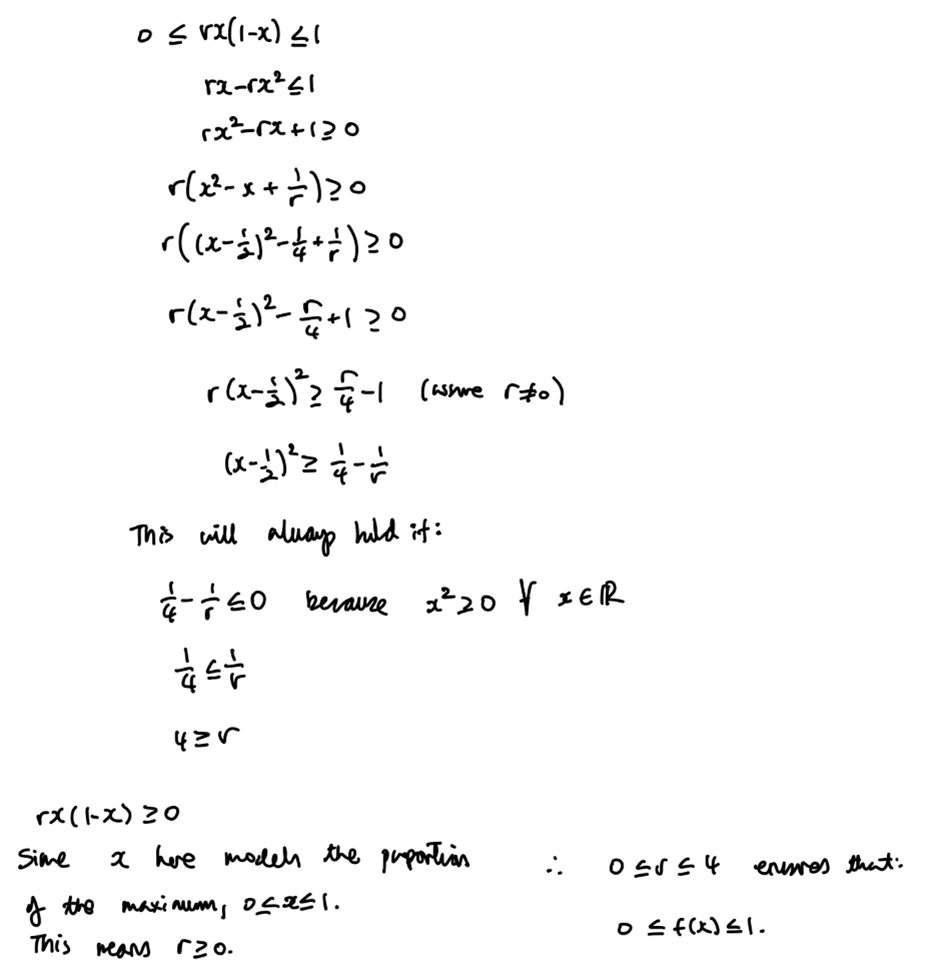Since the input of the next iteration is output of the previous iteration, bounds must be placed on the growth rate for the model to make sense. However, this seems a bit strange to me as I do not know why simply changing how the input variable is inputted results in bounds on the growth rate which is a meaningful variable in this context. A question to further ponder.

As well as this, I explored observation 2.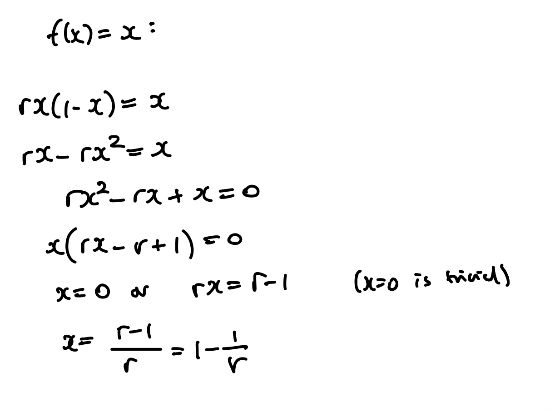This is effectively an oscillation about 1 point. However, to consider oscillation about $n$ points the equation $f^n\left(x\right)=x$ would need to be solved. This is equivalent to saying what value of $x$ would equal the value found when $f(x)$ is applied $n$ times to $x.$

Joshua and Louis did some more research about the logistic map and fractals.

Joshua wrote:

After attending the webinar 'Maths Makes a Difference - Making Predictions in an Uncertain World', I decided to further explore Chaos theory and logistic maps.

Since exploring the mathematics of logistic maps, I have developed my understanding of the meaning behind the constants and terms involved - in the logistic map of a population $x_{n+1}=kx_n(1-x_n),$ $k$ represents fertility, $x_n$ represents the existing population (as a proportion of the maximum population) and $x_{n+1}$ represents next year's population (as a proportion of the maximum population). Obviously, fertility is involved in the multiplication as next year's population depends on how fertile the existing population is, but the reason $x_n (1-x_n)$ is involved in the equation is because $x_n$ represents life, and $1-x_n$ represents death. If the population is currently large, it is expected to be large next year, but if it is too large, it dies out due to increased competition for food and hence starvation - multiplying $x_n (1-x_n)$ accounts for both scenarios, as too large a value of $x_n$ will mean $1-x_n$ is very small.

If the $k$ value (of fertility) is between $0$ and $1,$ the population dies out - if you iterate with any starting value for existing population, and the fertility is below $1,$ the iteration will eventually converge at $0.$

If the $k$ value is between approximately $1$ and $3,$ the population is stable - it eventually converges at a point where the population is living and dying at the same rate. However, if the $k$ value exceeds approximately $3,$ the population oscillates between different values - for instance, at $k =3.5,$ there is a four-year cycle where the population alternates between four different values.

At some point between $k = 3.5$ and $k = 4$, there is the onset of chaos. In mathematics, chaos means something is extremely sensitive to certain conditions. In this population model, beyond a certain value of $k,$ a tiny change in $k$ can lead to massively different behaviour. This makes the population model far less useful, as beyond this point, it is hard to predict population patterns.

The image below is a bifurcation diagram (which is simply a diagram/model where the line splits in two), you can see how after around $k = 3.6,$ the line just melts away into chaos. However, before this point, when the line is oscillating, the widths of each cycle (two cycle, then four cycle, then eight cycle, etc.) have a regular behaviour. Interestingly, each time, they are about $4.67$ times smaller than the previous width - this means the widths are part of a geometric series. This value of approximately $4.67$ is named the 'Feigenbaum constant'. Also, this population model can be referred to as a fractal, as it is self-similar and infinitely complex.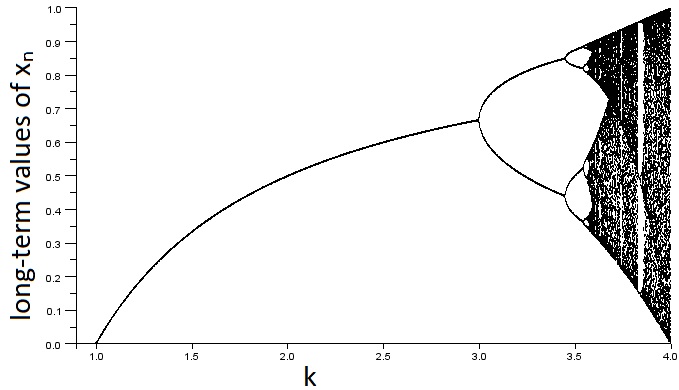Basti SchneiderBifurcation diagram logistic map lambda 0 to 4 (axis labels added by NRICH)

Louis gave some more information about the Feigenbaum constant:

A physicist Mitchell Feigenbaum discovered the constant that is now named after him, $4.669.$ By looking at when the bifurcations occurred, he examined the ratio of the width of one bifurcation and the proceeding bifurcation closed in on that number, $4.669.$ The fact that it is not derived from another constant means it is itself a fundamental constant of nature.

Louis and Joshua explained what the Mandelbrot set is and how it is related to the logistic map. Joshua wrote:

Moreover, I have recently discovered that this model links to the Mandelbrot set - after the onset of chaos, it becomes a one-dimensional Mandelbrot set. The Mandelbrot set shows the behaviour of $0$ under the iteration of $f_c,$ where $f_c (z)=z^2+c.$ This means setting the starting value of $z$ as $0,$ and choosing the value of $c.$ If the values of the iterations [do not] get arbitrarily large, this means the distance is bounded - it should never get larger than $2.$ [The values of $c$ for which the distance is bounded] (never get larger than $2$) are part of the Mandelbrot set.

If you check out the image below, you will see the Mandelbrot set. Then, when you look at the bifurcation diagram, you may be able to see that the onset of chaos looks like a one-dimensional version of the Mandelbrot set. It took me a while to see it, but even if you can't, it is nice to appreciate that two seemingly-unrelated topics can link so perfectly together - the beauty of mathematics.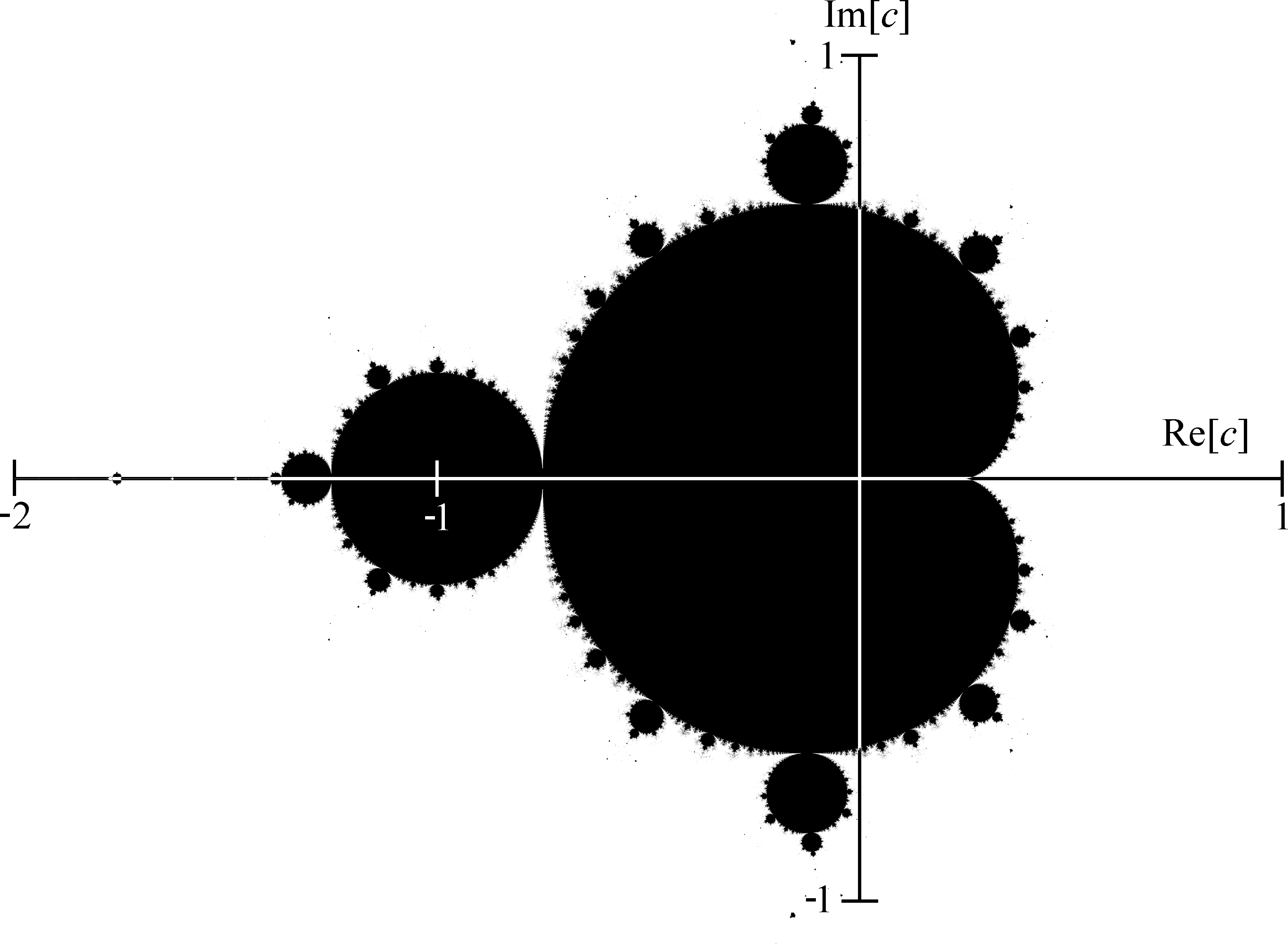Louis also gave some other examples:

In the real world, the logistic map and Feigenbaum's constant pop up frequently. Examples include fluid convection, rabbit heartbeats in fibrillation and even the dripping of water from your kitchen tap.

Manav did some more research into the logistic map and chaos theory:

Investigation: the logistic map and chaos theory

Aim: to discover how features of the logistic map and chaotic behaviour may be seen in real life.

Prior knowledge:
For $2\lt k\lt3$, $x_n$ oscillated between 2 values before tending to $\frac{k-1}{k}$. For $3\lt k\lt3.4,$ $x_n$ permanently oscillates about 2 values. From $3.4\lt k\lt3.6,$ $x_n$ oscillates about an increasing number of values, after which there is the onset of chaos. A period of stability occurs at $3.82\lt k\lt3.84.$ Small changes in $x_0$ seem to drastically influence value $x_n.$

Research:
It became apparent that the number of values around which $x_n$ oscillated was simply an exponential sequence 2, 4, 8 etc which occurred when the graph of $k$ and $x_n$ bifurcates at intervals. The time period between subsequent bifurcations follows the ratio of the Feigenbaum constant (4.669”¦).

The most intriguing follow-up was that similar chaotic behaviour can be seen in patients with arrhythmia (irregular heartbeat). Chaos has been found in the breakup of spiral and scroll waves, which are precursors to cardiac fibrillation. Perhaps this is linked to the logistic map: maybe the improper functioning of the sinoatrial node leads to minor imprecisions in coordinating heartbeat which gets amplified into displaying chaotic behaviour, similar to minor differences in parameter $x_0$ being magnified by exponential bifurcations in the logistic map resulting in chaos.

This phenomenon is the Butterfly Effect, where a system is highly sensitive to initial conditions. [It] has been witnessed by the meteorologist Lorenz who rounded off one variable in a simulator of weather patterns from 0.506127 to 0.506 and noticed a large difference in the outcome, prompting the suggestion that even one flap of a butterfly's wings could alter wind pressure to the extent that a tornado could form far away, and that forecasting the future is near impossible given that seemingly insignificant differences in parameters result in wildly dissimilar outcomes. Perhaps treatments could be developed that involve correcting these 'parameters' that determine heartbeat to induce a stable, non-chaotic outcome. Scientists were able to correct arrhythmia with an alternative method in rabbits by administering electrical stimuli to the heart at irregular times determined by chaos theory, converting it into periodic beating. Could similar theory be applied to influencing weather patterns for food security and agriculture; could the uncontrolled growth of cancerous cells display this chaotic behaviour and, by extension, could chaos theory be used to develop a cure?

Citations:
1) Weiss, J., Garfinkel, A., Karagueuzian, H., Qu, Z. and Chen, P., 2021. Chaos and the Transition to Ventricular Fibrillation : A New Approach to Antiarrhythmic Drug Evaluation. [online] Ahajournals.org. Available at: https://www.ahajournals.org/reader/content/165b7ae510e/10.1161/01.CIR.99.21.2819/format/epub/EPUB/xhtml/index.xhtml#R26> [Accessed 3 February 2021].
2) Dizikes, P., 2021. When the Butterfly Effect Took Flight. [online] MIT Technology Review. Available at: https://www.technologyreview.com/2011/02/22/196987/when-the-butterfly-effect-took-flight [Accessed 4 February 2021].
3) Garfinkel, A., Spano, M. L., Ditto, W. L., & Weiss, J. N. (1992). Controlling cardiac chaos. Science (New York, N.Y.), 257(5074), 1230-1235. https://doi.org/10.1126/science.1519060

Saanya researched the maths in weather forecasting:

Weather Forecasting and Chaos Theory

Vilhelm Bjerknes was one of the first mathematicians to consider the possibility of using mathematics in weather forecasting, in the late 19th century. It is now used globally as a tool for meteorologists, especially in computer algorithms, as there is too much data for a single human to process. Supercomputers are used to collect and observe data involving temperature, pressure, wind, tides, etc. which allow weather prediction models to then be formed from this. This is still not necessarily 100% accurate because of the theory of chaotic behaviour. Edward Lorenz, who discovered the atmosphere is chaotic, stated that it was actually impossible to forecast accurately for a duration of more than two weeks, as even a small error in initial conditions could create a whole new simulation. Modern forecasting models use a process called ensemble forecasting where the simulation is run several times each time with slightly different initial conditions and then averaged to find the most likely forecast. This can be done by constructing a grid to divide the atmosphere up. There are several different versions of this grid. However, in doing this, the atmosphere can be discretized, meaning researchers can get a finite number of quantitative values that can then be used in calculations over qualitative data that is much more difficult to interpret.

 Eminger S. The History of Weather Forecasting. Available from : https://mathshistory.st-andrews.ac.uk/HistTopics/Weather_forecasts/#3.1 [Accessed 2nd February 2021].

 NOAA Headquarters. The mathematics of weather prediction. Available from : https://phys.org/news/2016-05-mathematics-weather.html [Accessed 2nd February 2021].

Andrei from King's College London Maths School in England explored another application where maths is useful - the stock market:

The stock market is the aggregation of buyers and sellers of stocks, these stocks are equities in companies and this market plays part in a huge community and is used by investors and the populations that use it to make their livelihoods. The whole method associated with being successful in stocks is the ability to predict the fluctuations, dips and peaks in price of equities and decide in selling or buying certain stock in hope of turning a profit.

This idea of prediction and probability brings in the prospect of utilizing mathematical and statistical methods in order to increase your probability of success to its limit. There are many viable systems and practices that seem plausible such as Gaussian Laws which calculates random fluctuations of uncorrelated entities. This sounds ideal for playing the undulating stock market. However, all stock transactions are correlated, an influx of buys in one company causes the stock to rise exponentially.

Given this, we can instead use Power Laws, these laws calculate how the change in one quantity affects another.   Using these Laws, we can then proceed to calculate standard deviations for certain stocks and help traders identify the risk of certain companies and [identify the ones] which give the general highest probability of turning a profit.

One more statistical practice we can use is Quantitative Analysis, studying how amounts or quantities relate to each other and can help develop a model of the stock market helping trading houses. All these methods are accurate to some extent, however they come with many limitations and drawbacks.

Samuel from The Judd School in the UK explored the mathematical concept of Chaos further: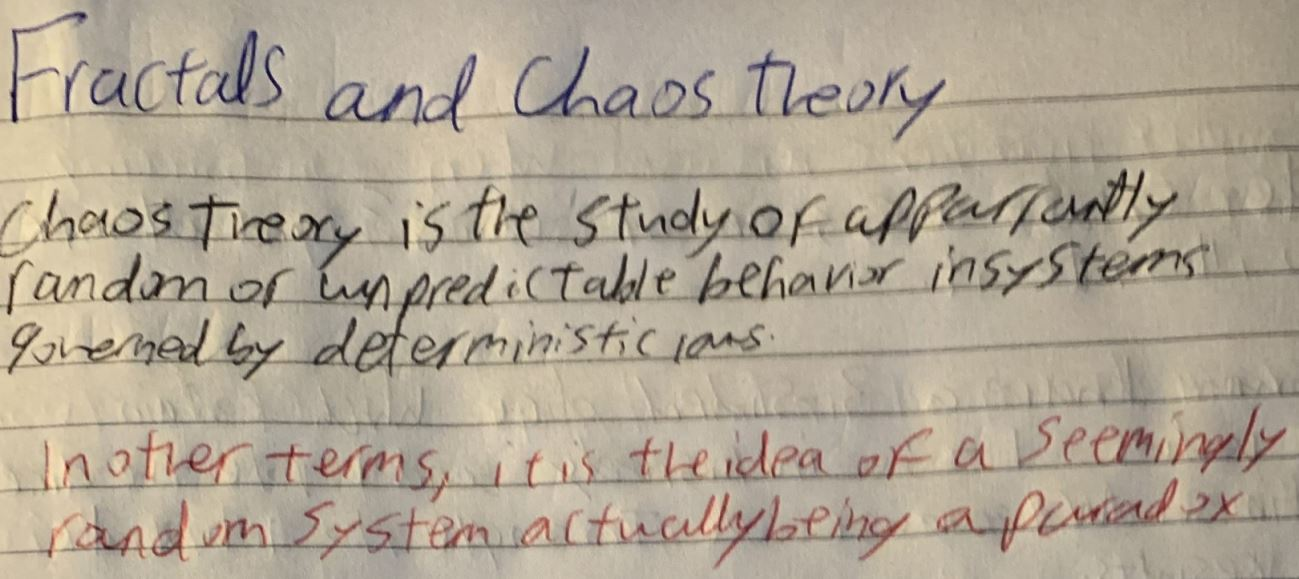a paradox in that the seemingly random system actually arose from an orderly set of rules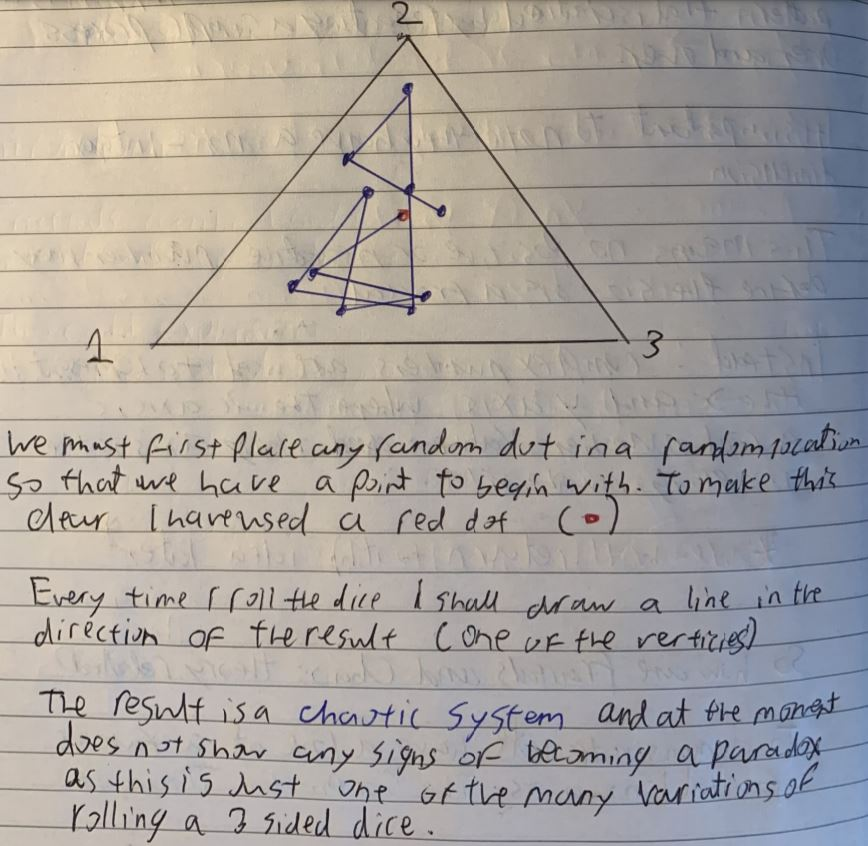In order to get this result, the distance that the dot moves each step must be controlled. The dot moves towards a vertex so that the distance between the point and the vertex is halved.

Can you see how this works? Try starting from any of the grey sections, and moving the point towards each of the vertices so that the distance between the dot and the vertex is halved. Will you always land in a grey section? What if you started with the dot in a white section?